# What in god’s name is Gradient Descent?

Gradient Descent is a very generic optimization algorithm capable of finding optimal solutions to a wide range of problems. The general idea (…) is to tweak parameters iteratively in order to minimize a cost function.

## What is a Cost Function?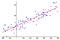Our linear model (red) generalising to all the data available (blue dots). Wikipedia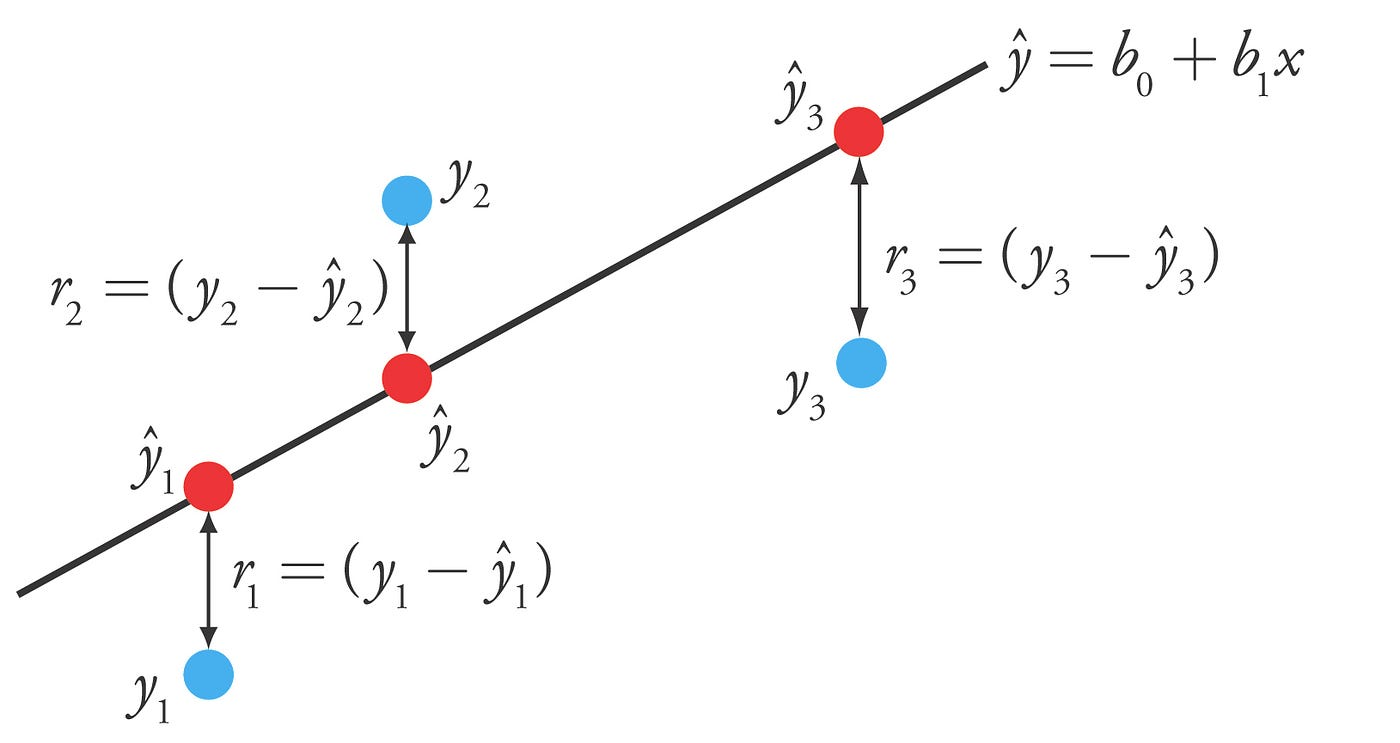Difference or distance between the predicted value (red) and the actual value (blue). Source

## Gradient Descent: Minimising the Cost Function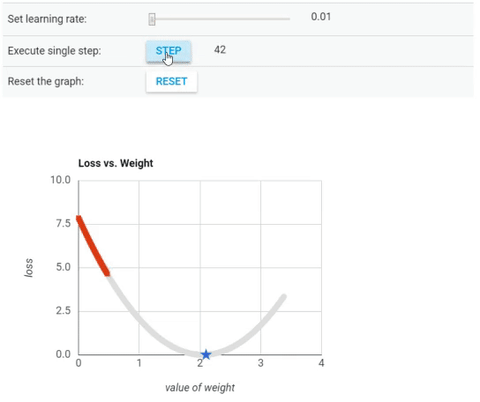Cost Function vs Value of Weight (parameter θ). Learning Rate = 0.10. Source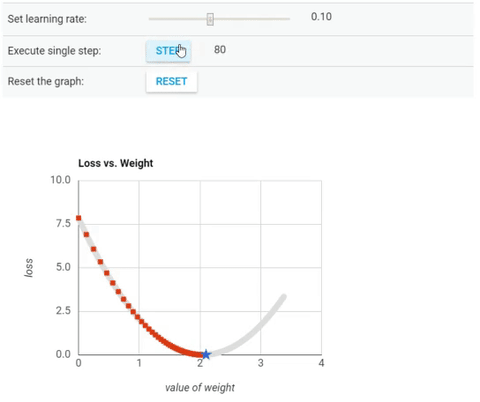Cost Function vs Value of Weight (parameter θ). Learning Rate = 1. Source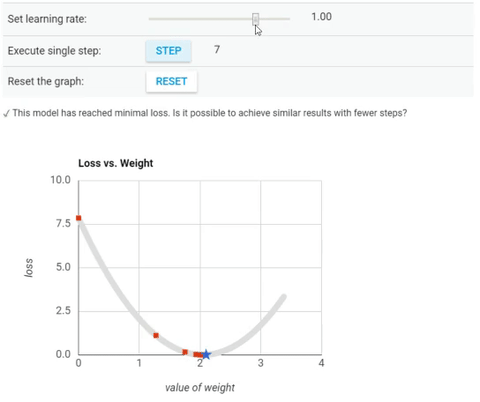Cost Function vs Value of Weight (parameter θ). Learning Rate = 1.6. Source

Written by